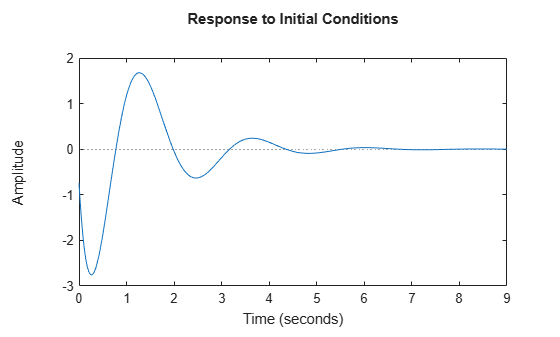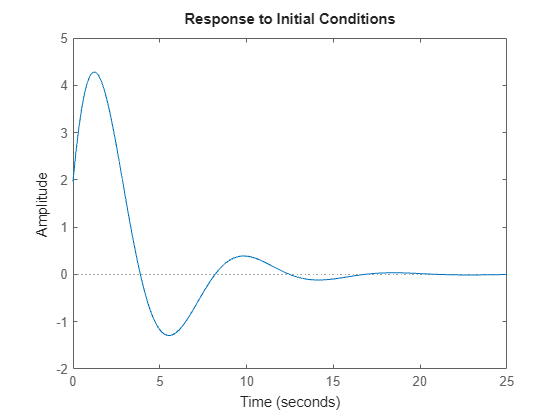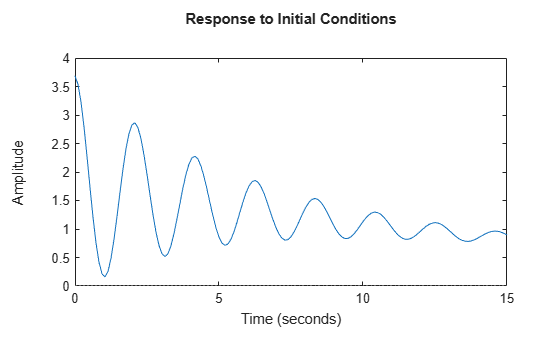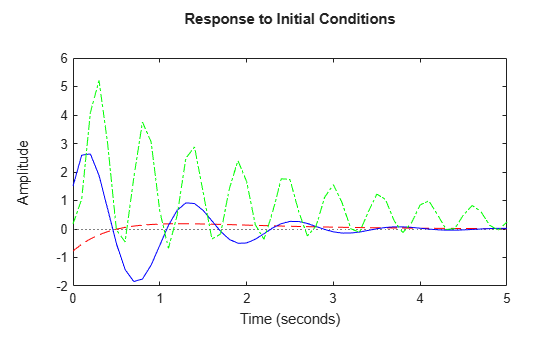# initial

System response to initial states of state-space model

## Syntax

``initial(sys,x0)``
``initial(sys,x0,tFinal)``
``initial(sys,x0,t)``
``initial(sys,x0,t,p)``
``initial(sys,{x0,p0},t,p)``
``initial(sys1,sys2,...,sysN,x0,___)``
``initial(sys1,LineSpec1,...,sysN,LineSpecN,x0,___)``
``y = initial(sys,x0,t)``
``y = initial(sys,x0,t,p)``
``y = initial(sys,{x0,p0},t,p)``
``[y,tOut,x] = initial(sys,x0)``
``[y,tOut,x,pOut] = initial(sys,x0,t,p)``

## Description

For state-space and sparse state-space models, `initial` computes the unforced system response y to initial states x0.

• Continuous time:

`$\begin{array}{cc}\stackrel{˙}{x}=Ax,& x\left({t}_{0}\right)={x}_{0}\\ y=Cx& \end{array}$`
• Discrete time:

`$\begin{array}{cc}x\left[k+1\right]=Ax\left[k\right]& x\left[{k}_{0}\right]={x}_{0}\\ y=Cx\left[k\right]& \end{array}$`

For linear time-varying or linear parameter-varying state-space models, `initial` computes the response with initial state x0, initial parameter p0 (LPV models), and input held to the offset value (u(t) = u0(t) or u(t) = u0(t,p). This corresponds to the initial condition response of the local linear dynamics.

### Initial Response Plots

example

````initial(sys,x0)` plots the unforced system response to initial states of a state-space (`ss`) model `sys` with an initial condition on the states specified by the vector `x0`:The state-space model `sys` can be continuous-time or discrete-time, and SISO or MIMO. For MIMO state-space systems, the plot displays the responses for the outputs of each channel. `initial` automatically determines the time steps and duration of the simulation based on the system dynamics.```

example

````initial(sys,x0,tFinal)` plots the simulated response from `t = 0` to the final time ```t = tFinal```. The function uses system dynamics to determine the intervening time steps.```

example

````initial(sys,x0,t)` plots the response at the times that you specify in the vector `t`. ```
````initial(sys,x0,t,p)` also specifies the parameter trajectory `p` for `lpvss` models. ```

example

````initial(sys,{x0,p0},t,p)` also specifies the initial parameter value `p0` and parameter trajectory `p` for LPV models. `p0` is required for implicit parameter trajectories.```

example

````initial(sys1,sys2,...,sysN,x0,___)` plots the response of multiple dynamic systems on the same plot. All systems must have the same number of inputs and outputs. You can use multiple dynamic systems with any of the previous input-argument combinations.```

example

````initial(sys1,LineSpec1,...,sysN,LineSpecN,x0,___)` specifies a color, line style, and marker for each system in the response plot. You can use `LineSpec` with any of the previous input-argument combinations. When you need additional plot customization options, use `initialplot` instead.```

### Initial Response Data

````y = initial(sys,x0,t)` returns the initial response `sys` at the times specified in the vector `t`. This syntax does not draw a plot.```
````y = initial(sys,x0,t,p)` also specifies the parameter trajectory `p` for LPV models.```

example

````y = initial(sys,{x0,p0},t,p)` also specifies the initial parameter value and parameter trajectory `p` for LPV models. `p0` is required for implicit parameter trajectories.```

example

````[y,tOut,x] = initial(sys,x0)` returns the output response `y`, the time vector `tOut` and the state trajectories `x`. The array `y` has as many rows as time samples (length of `tOut`) and as many columns as outputs. Similarly, `x` has `length(tOut)` rows and as many columns as states.```
````[y,tOut,x,pOut] = initial(sys,x0,t,p)` also returns parameter trajectories `pOut`, when `sys` is an `lpvss` model.```

## Examples

collapse all

For this example, generate a random state-space model with 5 states and create the plot for the system response to the initial states.

```rng("default") sys = rss(5); x0 = [1,2,3,4,5]; initial(sys,x0)```Plot the response of the following state-space model:

`$\begin{array}{rcl}\left[\begin{array}{l}{\underset{}{\overset{˙}{x}}}_{1}\\ {\underset{}{\overset{˙}{x}}}_{2}\end{array}\right]& =& \left[\begin{array}{cc}-0.5572& -0.7814\\ 0.7814& 0\end{array}\right]\left[\begin{array}{l}{x}_{1}\\ {x}_{2}\end{array}\right]\\ y& =& \left[\begin{array}{cc}1.9691& 6.4493\end{array}\right]\left[\begin{array}{l}{x}_{1}\\ {x}_{2}\end{array}\right].\end{array}$`

Take the following initial condition:

`$x\left(0\right)=\left[\begin{array}{l}1\\ 0\end{array}\right].$`

```a = [-0.5572, -0.7814; 0.7814, 0]; c = [1.9691 6.4493]; x0 = [1 ; 0]; sys = ss(a,[],c,[]); initial(sys,x0)```Consider the following two-input, two-output dynamic system.

`$sys\left(s\right)=\left[\begin{array}{cc}0& \frac{3s}{{s}^{2}+s+10}\\ \frac{s+1}{s+5}& \frac{2}{s+6}\end{array}\right].$`

Convert the `sys` to state-space form since initial condition response plots are supported only for state-space models.

```sys = ss([0, tf([3 0],[1 1 10]) ; tf([1 1],[1 5]), tf(2,[1 6])]); size(sys)```
```State-space model with 2 outputs, 2 inputs, and 4 states. ```

The resultant state-space model has four states. Hence, provide an initial condition vector with four elements.

`x0 = [0.3,0.25,1,4];`

Create the initial condition response plot.

`initial(sys,x0);`The resultant plot contains two subplots - one for each output in `sys`.

For this example, examine the initial condition response of the following zero-pole-gain model and limit the plot to `tFinal` = 15 s.

First, convert the `zpk` model to an `ss` model since `initial` only supports state-space models.

```sys = ss(zpk(-1,[-0.2+3j,-0.2-3j],1)*tf([1 1],[1 0.05])); tFinal = 15; x0 = [4,2,3];```

Now, create the initial conditions response plot.

`initial(sys,x0,tFinal);`For this example, plot the initial condition responses of three dynamic systems.

First, create the three models and provide the initial conditions. All the models should have the same number of states.

```rng('default'); sys1 = rss(4); sys2 = rss(4); sys3 = rss(4); x0 = [1,1,1,1];```

Plot the initial condition responses of the three models using time vector `t` that spans 5 seconds.

```t = 0:0.1:5; initial(sys1,'r--',sys2,'b',sys3,'g-.',x0,t)```Extract the initial condition response data of the following state-space model with two states:

`$\begin{array}{rcl}\left[\begin{array}{l}{\underset{}{\overset{˙}{x}}}_{1}\\ {\underset{}{\overset{˙}{x}}}_{2}\end{array}\right]& =& \left[\begin{array}{cc}-0.5572& -0.7814\\ 0.7814& 0\end{array}\right]\left[\begin{array}{l}{x}_{1}\\ {x}_{2}\end{array}\right]\\ y& =& \left[\begin{array}{cc}1.9691& 6.4493\end{array}\right]\left[\begin{array}{l}{x}_{1}\\ {x}_{2}\end{array}\right].\end{array}$`

Use the following initial conditions:

`$x\left(0\right)=\left[\begin{array}{l}1\\ 0\end{array}\right].$`

```a = [-0.5572, -0.7814; 0.7814, 0]; c = [1.9691 6.4493]; x0 = [1 ; 0]; sys = ss(a,[],c,[]); [y,tOut,x] = initial(sys,x0);```

The array `y` has as many rows as time samples (length of `tOut`) and as many columns as outputs. Similarly, `x` has rows equal to the number of time samples (length of `tOut`) and as many columns as states.

For this example, extract the initial condition response data of a state-space model with 6 states, 3 outputs and 2 inputs.

First, create the model and provide the initial conditions.

```rng('default'); sys = rss(6,3,2); x0 = [0.1,0.3,0.05,0.4,0.75,1];```

Extract the initial condition responses of the model using time vector `t` that spans 15 seconds.

```t = 0:0.1:15; [y,tOut,x] = initial(sys,x0,t);```

The array `y` has as many rows as time samples (length of `tOut`) and as many columns as outputs. Similarly, `x` has rows equal to the number of time samples (length of `tOut`) and as many columns as states.

For this example, consider `lpvHCModel.m` that defines the following model.

`$\stackrel{˙}{\mathit{x}}=-\left(\mathit{x}-{\mathit{x}}_{0}\left(\mathit{p}\right)\right)+\left(\mathit{u}-{\mathit{u}}_{0}\left(\mathit{p}\right)\right)$`

`$\mathit{y}={\mathit{y}}_{0}\left(\mathit{p}\right)+\left(1-{\mathit{p}}^{2}\right)\left(\mathit{x}-{\mathit{x}}_{0}\left(\mathit{p}\right)\right)$`

`$\left({\mathit{x}}_{0}\left(\mathit{p}\right),{\mathit{u}}_{0}\left(\mathit{p}\right),{\mathit{y}}_{0}\left(\mathit{p}\right)\right)=\left({\mathrm{tanh}}^{-1}\left(\mathit{p}\right),{\mathrm{tanh}}^{-1}\left(\mathit{p}\right),\mathit{p}\right)$`

Use `lpvss` to construct this LPV plant. Since ${\mathrm{tanh}}^{-1}\left(\mathit{p}\right)$ is infinite for $|\mathit{p}|=1$, clip $\mathit{p}$ to the range $\left[-{\mathit{p}}_{\mathrm{max}},{\mathit{p}}_{\mathrm{max}}\right]$ to stay away from singularity.

```pmax = 0.99; vSys = lpvss('p',@(t,p) lpvHCModel(t,p,pmax),'StateName','x');```

You can compute the initial response for this model along a trajectory $\mathit{p}\left(\mathit{t}\right)$.

Define $\mathit{p}\left(\mathit{t}\right)$ implicitly as a function $\mathit{F}\left(\mathit{t},\mathit{x},\mathit{u}\right)$ of time $\mathit{t}$, state $\mathit{x}$, and input $\mathit{u}$. For this model, use $\mathit{p}\left(\mathit{t}\right)=\mathit{y}\left(\mathit{t}\right)=\mathrm{tanh}\left(\mathit{x}\right)$.

```pinit = 0.5; xinit = 0.5; t= linspace(0,1,100); p = @(t,x,u) tanh(x); initial(vSys,{xinit,pinit},t,p);````initial` computes the response with initial state, initial parameter, and input held to offset value ${\mathit{u}}_{0}\left(\mathit{t},\mathit{p}\right)$.

## Input Arguments

collapse all

Dynamic system, specified as a SISO or MIMO dynamic system model or array of dynamic system models. You can only use state-space models of the following types:

• Continuous-time or discrete-time numeric `ss` models.

• Generalized or uncertain LTI models such as `genss` or `uss` models. (Using uncertain models requires Robust Control Toolbox™ software.)

• For tunable control design blocks, the function evaluates the model at its current value for both plotting and returning response data.

• For uncertain control design blocks, the function plots the nominal value and random samples of the model. When you use output arguments, the function returns response data for the nominal model only.

• Sparse state-space models such as `sparss` and `mechss` models. You must specify final time `tFinal` for sparse state-space models.

• Linear time-varying (`ltvss`) and linear parameter-varying (`lpvss`) models.

If `sys` is an array of models, the function plots the responses of all models in the array on the same axes.

End time to compute the response, specified as a positive scalar value. `initial` simulates the response from `t = 0` to `t = ``tFinal`.

• For continuous-time systems, the function determines the step size and number of points automatically from system dynamics. Express `tFinal` in the system time units, specified in the `TimeUnit` property of `sys`.

• For discrete-time systems, the function uses the sample time of `sys` as the step size. Express `tFinal` in the system time units, specified in the `TimeUnit` property of `sys`.

• For discrete-time systems with unspecified sample time (```Ts = -1```), `initial` interprets `tFinal` as the number of sampling periods to simulate.

Time vector at which to compute the response, specified as a vector of positive scalar values. Express `t` in the system time units, specified in the `TimeUnit` property of `sys`.

• For continuous-time models, specify `t` in the form `Ti:dt:Tf`. To obtain the response at each time step, the function uses `dt` as the sample time of a discrete approximation to the continuous system.

• For discrete-time models, specify `t` in the form `Ti:Ts:Tf`, where `Ts` is the sample time of `sys`.

Line style, marker, and color, specified as a string or vector of one, two, or three characters. The characters can appear in any order. You do not need to specify all three characteristics (line style, marker, and color). For example, if you omit the line style and specify the marker, then the plot shows only the marker and no line. For more information about configuring this argument, see the `LineSpec` input argument of the `plot` function.

Example: `'r--'` specifies a red dashed line

Example: `'*b'` specifies blue asterisk markers

Example: `'y'` specifies a yellow line

Initial condition of the states, specified as a vector. `x0` must have the same length as the number of states in `sys`.

Parameter trajectory of the LPV model, specified as a matrix or a function handle.

• For exogenous or explicit trajectories, specify `p` as a matrix with dimensions N-by-Np, where N is the number of time samples and Np is the number of parameters.

Thus, the row vector `p(i,:)` contains the parameter values at the ith time step.

• For endogenous or implicit trajectories, specify `p` as a function handle of the form p = F(t,x,u) in continuous time and p = F(k,x,u) in discrete time that gives parameters as a function of time t or time sample k, state x, and input u. The initial parameter value `p0` is required for this input method.

Initial value of the parameters of LPV model, specified as a vector. `p0` must have the same length as the number of parameters in `sys`.

## Output Arguments

collapse all

Response data, returned as an array.

• For SISO systems, `y` is a column vector of the same length as `t` (if provided) or `tOut` (if you do not provide `t`).

• For single-input, multi-output systems, `y` is a matrix with as many rows as there are time samples and as many columns as there are outputs. Thus, the jth column of `y`, or `y(:,j)`, contains the response of from the input to the jth output.

• For MIMO systems, the dimensions of `y` are then N-by-Ny, where:

• N is the number of time samples.

• Ny is the number of system outputs.

Times at which response is computed, returned as a vector. When you do not provide a specific vector `t` of times, `initial` chooses this time vector based on the system dynamics. The times are expressed in the time units of `sys`.

State trajectories, returned as an array. `x` contains the evolution of the states of `sys` at each time in `t` or `tOut`. The dimensions of `x` are N-by-Nx, where:

• N is the number of time samples.

• Nx is the number of states.

Parameter trajectories, returned as an array. When `sys` is a linear-parameter varying (`lpvss`) model, `pOut` contains the evolution of the parameters of `sys`. The dimensions of `pOut` are N-by-Np, where:

• N is the number of time samples.

• Np is the number of parameters.

## Version History

Introduced before R2006a